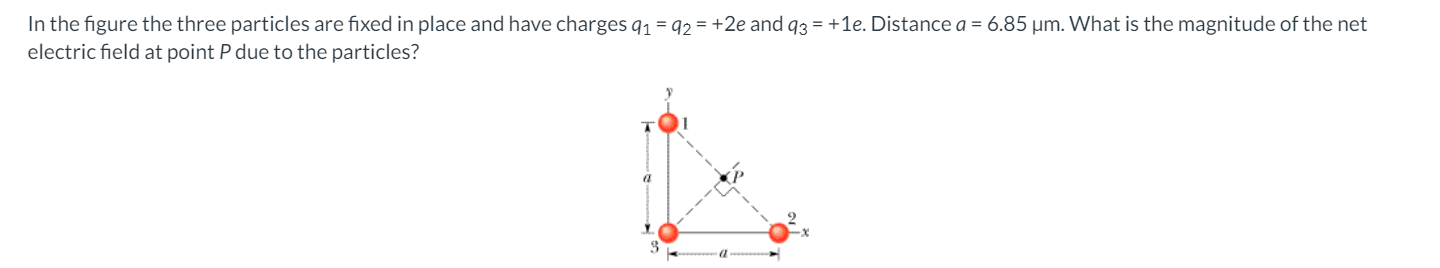Home / Answered Questions / Other / question-34-part-1-part-two-a-thin-nonconducting-rod-with-a-uniform-distribution-of-positive-charge--aw942

# (Solved): Question 34) Part 1) Part Two: A Thin Nonconducting Rod With A Uniform Distribution Of Positive Char...

Question 34)

Part 1)Part Two:

A thin nonconducting rod with a uniform distribution of positive charge Q is bent into a circle of radius R (see the figure). The central perpendicular axis through the ring is a z axis, with the origin at the center of the ring. What is the magnitude of the electric field due to the rod at (a) z = 0 and (b) z = âˆž? (c) In terms of R, at what positive value of z is that magnitude maximum? (d) If R = 2.31 cm and Q = 4.07 Î¼C, what is the maximum magnitude?

In the figure the three particles are fixed in place and have charges 91 = 92 = +2e and 93 = +1e. Distance a = 6.85 um. What is the magnitude of the net electric field at point P due to the particles? -

We have an Answer from Expert